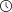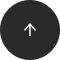- 3 minutes read

# Why Window Functions Are Not Allowed in WHERE Clauses

Window functions are extremely powerful, but there are a lot of nuances and little details you need to learn about using window functions in order to use them correctly and efficiently. A fairly common question SQL users have is why window functions are not allowed in WHERE.

TL;DR: You can use window functions in `SELECT` and `ORDER BY`. You cannot use window functions in `WHERE`, `GROUP BY`, or `HAVING`. Use a subquery or a `WITH` query to get around this limitation.

Read on to find out why.

### Using ROW_NUMBER() in a WHERE Clause

Here is an example. The query below tries to find salespeople who made the sale with the highest amount for each product. It seems like a perfect use for the `ROW_NUMBER()` function. Let’s try: we want to number the rows within each product, in the order from the highest to the lowest by sale amount. This is a typical attempt for using window functions in `WHERE`.

```SELECT id, product_id, salesperson_id, amount
FROM sale
WHERE 1 = row_number() over(PARTITION BY product_id ORDER BY amount DESC);
```

However, when we run the query, we get an error:

```ERROR:  window functions are not allowed in WHERE
LINE 3: WHERE 1 = row_number() over(PARTITION BY product_id ORDER BY amount DESC)
```

### Why Can’t I Use ROW_NUMBER() in WHERE?

You can’t use window functions in `WHERE`, because the logical order of operations in an SQL query is completely different from the SQL syntax. The logical order of operations in SQL is:

1. `FROM, JOIN`
2. `WHERE`
3. `GROUP BY`
4. aggregate functions
5. `HAVING`
6. window functions
7. `SELECT`
8. `DISTINCT`
9. `UNION/INTERSECT/EXCEPT`
10. `ORDER BY`
11. `OFFSET`
12. `LIMIT/FETCH/TOP`

This means that the filters in a `WHERE` clause are processed before window functions. At the time the `WHERE` clause is processed, the window functions would not have been computed yet. And if they are not yet computed, you can’t refer to them in `WHERE`.

This order of operations implies that you can only use window functions in `SELECT` and `ORDER BY`. That is, window functions are not accessible in `WHERE`, `GROUP BY`, or `HAVING` clauses. For this reason, you cannot use any of these functions in `WHERE`: `ROW_NUMBER()`, `RANK()`, `DENSE_RANK()`, `LEAD()`, `LAG()`, or `NTILE()`.

Is there a way around it? Fortunately, yes!

### How Do I Use Window Functions in a WHERE Clause Then?

The solution is to use either a subquery or a WITH query (aka Common Table Expression). Take a look at the following:

```WITH sales_numbered AS (
SELECT id, product_id, salesperson_id, amount,
row_number() over(PARTITION BY product_id ORDER BY amount DESC) AS rn
FROM sale
)
SELECT id, product_id, salesperson_id, amount
FROM sales_numbered
WHERE rn = 1;
```

In the `WITH` query, we select the columns from the table `sales` and compute the row number value for each row. In the main query, we use the computed row number value, `rn`, to filter the rows with the row number equal to 1.

### Why Can’t I Use Window Functions in WHERE?

You can’t use window functions in `WHERE`, because they would not have been evaluated yet at the time the `WHERE` filters are processed. You can use window functions only in `SELECT` and `ORDER BY`.

If you would like to learn more about window functions in SQL, try our interactive “Window Functions” course. We teach window functions syntax on real world examples, discuss common mistakes, and explain little caveats that will make your work more effective.